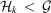International Tables for Crystallography (2011). Vol. A1, ch. 2.1, pp. 72-96   | 1 | 2 | https://doi.org/10.1107/97809553602060000797

## Contents

• 2.1. Guide to the subgroup tables and graphs  (pp. 72-96)
• 2.1.1. Contents and arrangement of the subgroup tables  (p. 72) | html | pdf |
• 2.1.2. Structure of the subgroup tables  (pp. 72-76) | html | pdf |
• 2.1.2.1. Headline  (p. 72) | html | pdf |
• 2.1.2.2. Data from IT A  (pp. 72-73) | html | pdf |
• 2.1.2.2.1. Generators selected  (p. 72) | html | pdf |
• 2.1.2.2.2. General position  (pp. 72-73) | html | pdf |
• 2.1.2.3. Specification of the setting  (p. 73) | html | pdf |
• 2.1.2.4. Sequence of the subgroup and supergroup data  (p. 73) | html | pdf |
• 2.1.2.5. Special rules for the setting of the subgroups  (pp. 74-76) | html | pdf |
• 2.1.2.5.1. Monoclinic subgroups  (p. 74) | html | pdf |
• 2.1.2.5.2. Subgroups with two origin choices  (pp. 74-75) | html | pdf |
• 2.1.2.5.3. Space groups with a rhombohedral lattice  (pp. 75-76) | html | pdf |
• 2.1.3. I Maximal translationengleiche subgroups ( t -subgroups)  (pp. 76-79) | html | pdf |
• 2.1.3.1. Introduction  (p. 76) | html | pdf |
• 2.1.3.2. A description in close analogy with IT A  (pp. 76-77) | html | pdf |
• 2.1.3.3. Basis transformation and origin shift  (pp. 77-79) | html | pdf |
• 2.1.4. II Maximal klassengleiche subgroups ( k -subgroups)  (pp. 79-82) | html | pdf |
• 2.1.4.1. General description  (p. 79) | html | pdf |
• 2.1.4.2. Loss of centring translations  (pp. 79-80) | html | pdf |
• 2.1.4.3. Enlarged unit cell  (pp. 80-82) | html | pdf |
• 2.1.4.3.1. Enlarged unit cell, index 2  (pp. 80-81) | html | pdf |
• 2.1.4.3.2. Enlarged unit cell, index 3 or 4  (pp. 81-82) | html | pdf |
• 2.1.5. Series of maximal isomorphic subgroups  (pp. 82-84) | html | pdf |
• 2.1.5.1. General description  (p. 82) | html | pdf |
• 2.1.5.2. Basis transformation  (p. 82) | html | pdf |
• 2.1.5.3. Origin shift  (p. 82) | html | pdf |
• 2.1.5.4. Generators  (pp. 82-83) | html | pdf |
• 2.1.5.5. Special series  (pp. 83-84) | html | pdf |
• 2.1.5.5.1. Monoclinic space groups  (p. 83) | html | pdf |
• 2.1.5.5.2. Trigonal space groups with rhombohedral lattice  (p. 83) | html | pdf |
• 2.1.5.5.3. Space groups with two origin choices  (pp. 83-84) | html | pdf |
• 2.1.6. The data for minimal supergroups  (pp. 84-85) | html | pdf |
• 2.1.6.1. Description of the listed data  (p. 84) | html | pdf |
• 2.1.6.2. I Minimal translationengleiche supergroups  (pp. 84-85) | html | pdf |
• 2.1.6.3. II Minimal non-isomorphic klassengleiche supergroups  (p. 85) | html | pdf |
• 2.1.7. Derivation of the minimal supergroups from the subgroup tables  (pp. 86-90) | html | pdf |
• 2.1.7.1. Determination of the non-isomorphic minimal k -supergroups by inverting the subgroup data  (p. 86) | html | pdf |
• 2.1.7.2. The isomorphic minimal supergroups  (pp. 86-87) | html | pdf |
• 2.1.7.3. Determination of one minimal t -supergroup by inverting the subgroup data  (pp. 87-88) | html | pdf |
• 2.1.7.4. Derivation of further minimal t-supergroups by using normalizers  (pp. 88-90) | html | pdf |
• 2.1.7.5. Derivation of the remaining minimal t -supergroups  (p. 90) | html | pdf |
• 2.1.8. The subgroup graphs  (pp. 90-96) | html | pdf |
• 2.1.8.1. General remarks  (pp. 90-91) | html | pdf |
• 2.1.8.2. Graphs for translationengleiche subgroups  (pp. 91-92) | html | pdf |
• 2.1.8.3. Graphs for klassengleiche subgroups  (pp. 92-93) | html | pdf |
• 2.1.8.4. Graphs for plane groups  (p. 93) | html | pdf |
• 2.1.8.5. Application of the graphs  (pp. 93-96) | html | pdf |
• References | html | pdf |
• Figures
• Fig. 2.1.7.1. In the left-hand diagram, there are i conjugate subgroupsofif [ i ] is the index ofin; in the right-hand diagram,is a normal subgroup of(p. 88) | html | pdf |
• Fig. 2.1.8.1. Contracted graph of the group–subgroup chains from(225) to one of those subgroups with index 12 which belong to the space-group type(12)  (p. 94) | html | pdf |
• Fig. 2.1.8.2. Complete graph of the group–subgroup chains from(225) to one representative belonging to those six(12) subgroups with index 12 whose monoclinic axes are along thedirections of(p. 94) | html | pdf |
• Fig. 2.1.8.3. Complete graph of the group–subgroup chains from(225) to, which is one representative of those three(12) subgroups with index 12 whose monoclinic axes are along thedirections of(p. 94) | html | pdf |
• Fig. 2.1.8.4. Complete graph of the group–subgroup chains from perovskite,, here high-temperature SrTiO 3 , to the four subgroups of type I 4/ mcm with their tetragonal axes in the c direction  (p. 96) | html | pdf |
• Fig. 2.1.8.5. Two different subgroups of P 4/ mmm , both of type I 4/ mcm , correspond to two kinds of distortions of the coordination octahedra of the perovskite structure  (p. 96) | html | pdf |